Shen

# Abaqus周期边界条件的设置

Mar 19, 2018 • 9 min read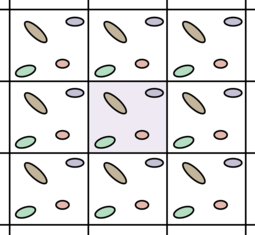周期性结构

• 在x方向上的边界上 $\Gamma_l$ 和 $\Gamma_r$ 存在应变载荷；
• 在y方向上的边界上 $\Gamma_b$ 和 $\Gamma_t$ 存在周期边界。

\begin{equation*} u_i-u_j=u_{Ref1}\\ v_i-v_j=v_{Ref1} \end{equation*}

\begin{equation*} u_k-u_l=u_{Ref2}\\ v_k-v_l=v_{Ref2} \end{equation*}

\begin{equation*} v_{Ref1}=\epsilon*(y_i-y_j)\\ v_{Ref2}=\epsilon*(y_k-y_l) \end{equation*}

• 确定两个参考点；
• 确定四个边界上的节点编号，分别储存在left，right，bottom和top四个数组中；
• 在对应的两个边界上例如left和right，以及bottom和top中，寻找距离最近的两个节点作为一对，设置边界条件。具体方法是采用双重循环，在left中任取一个节点M，然后遍历right中的所有节点，找到和节点M距离最近的节点，即为与之配对的节点N。bottom和top也采用类似操作；
• 在load模块中设置两个参考点的位移约束。

## 2D算例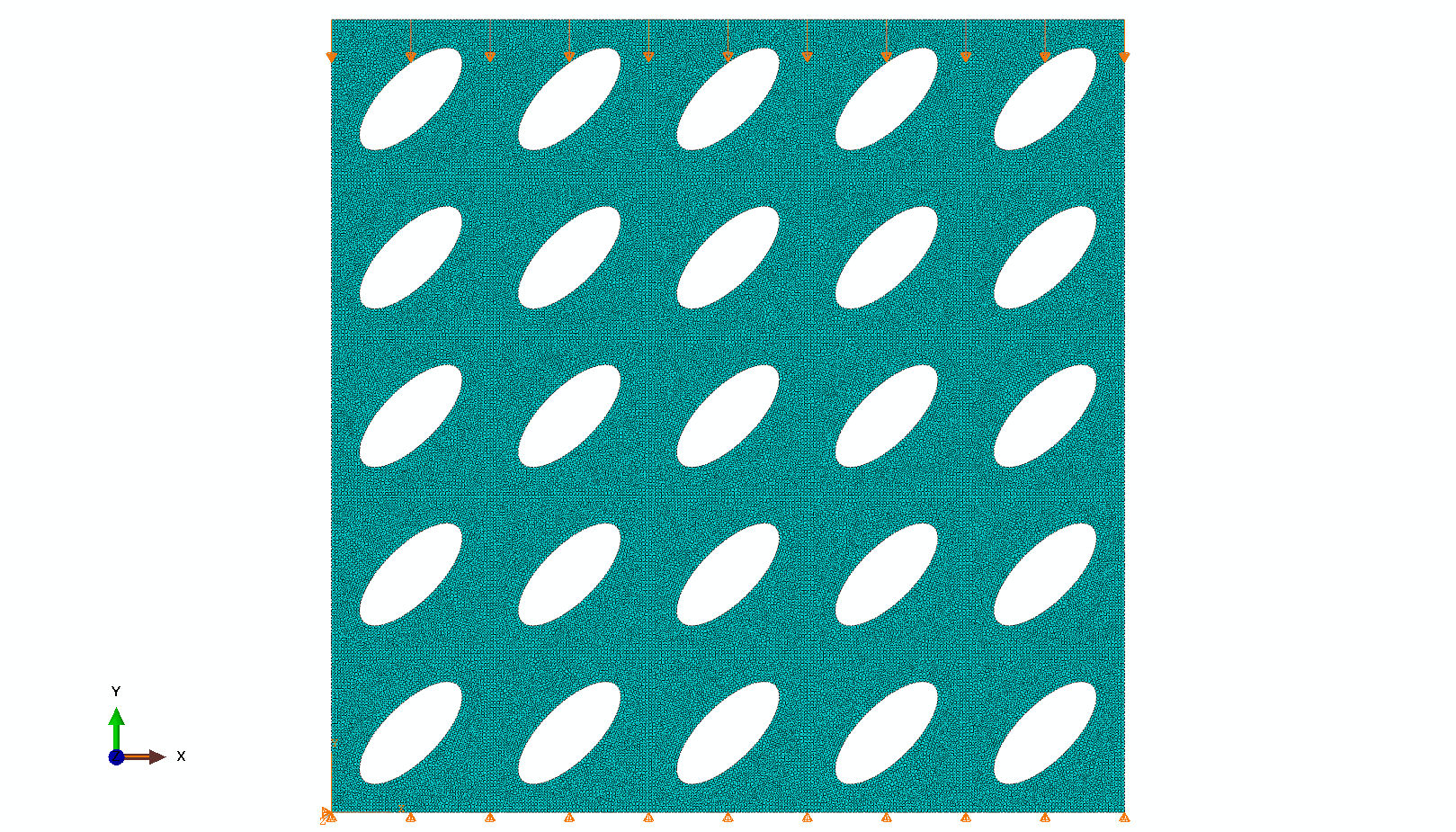2D周期性结构

### 整体模型计算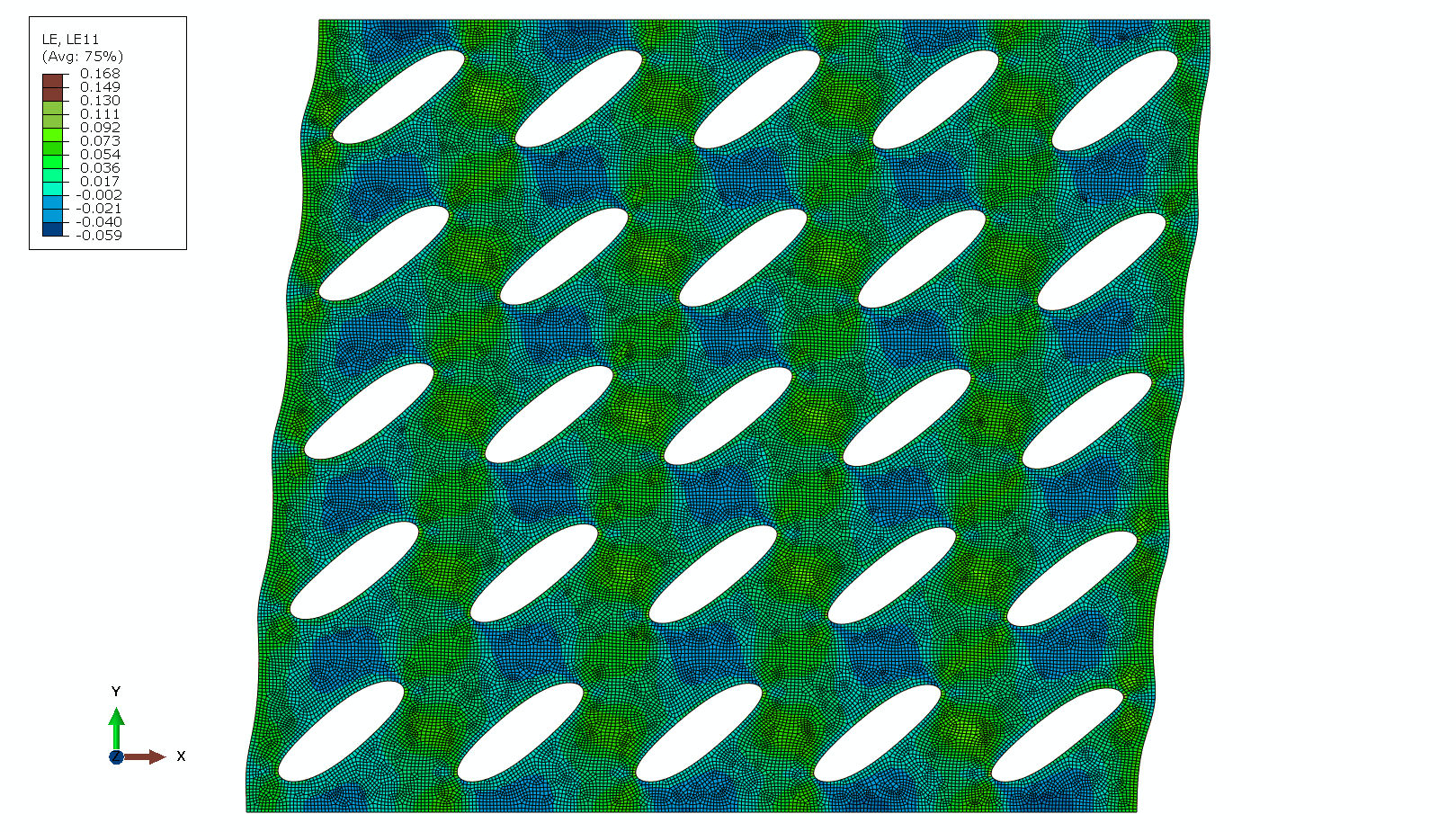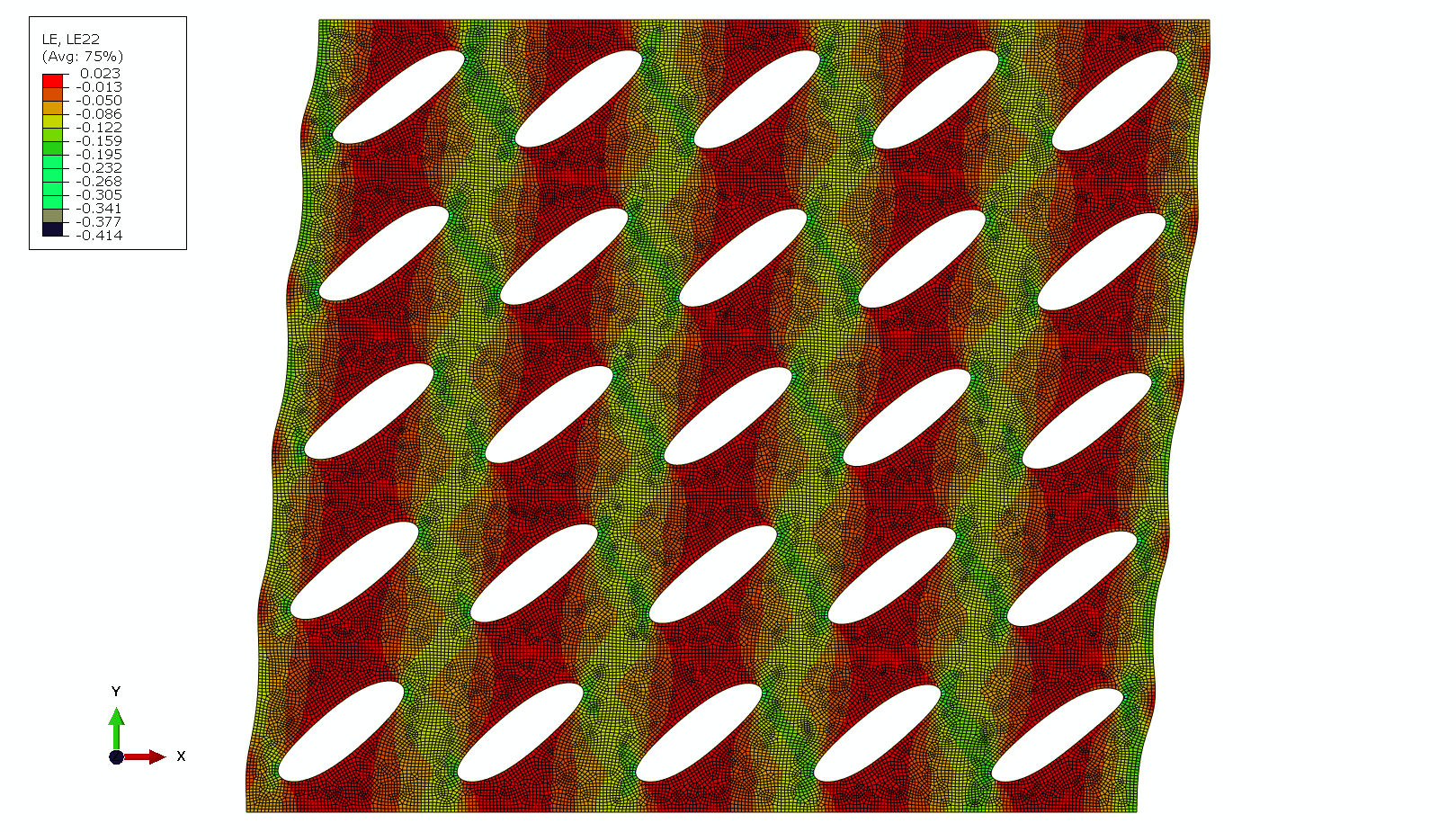整体结构的应变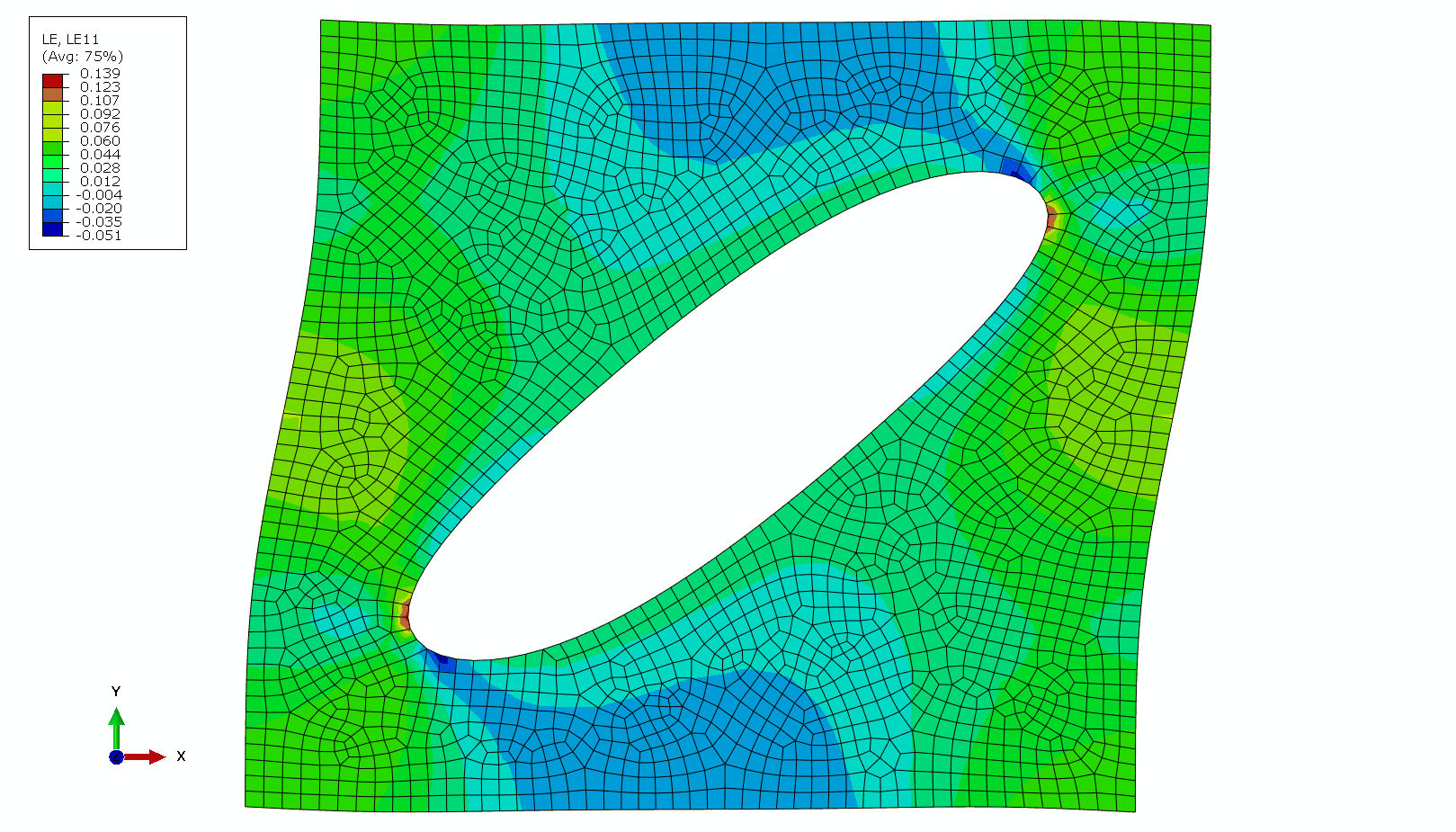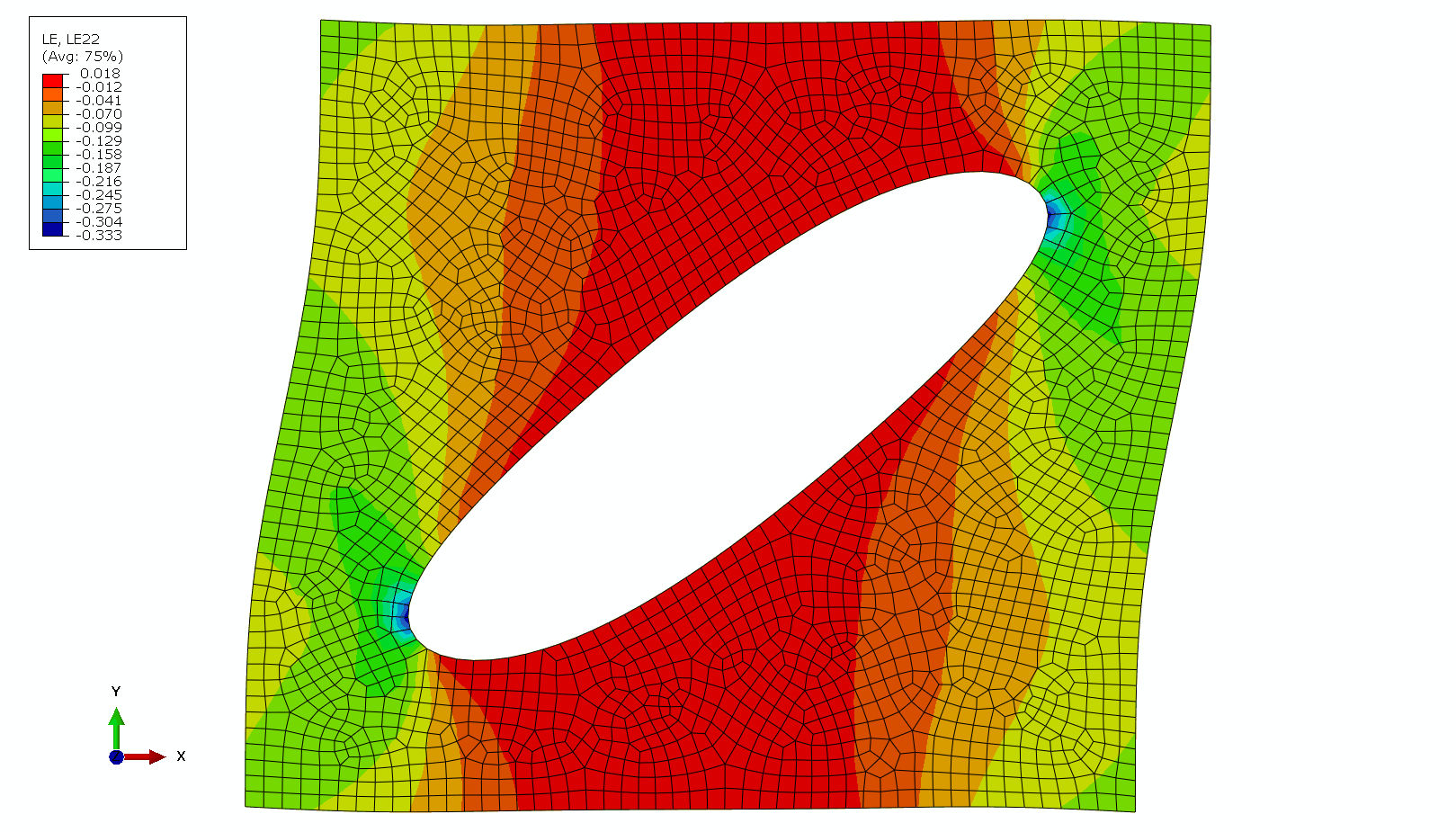中心单元的应变

### 周期边界模型计算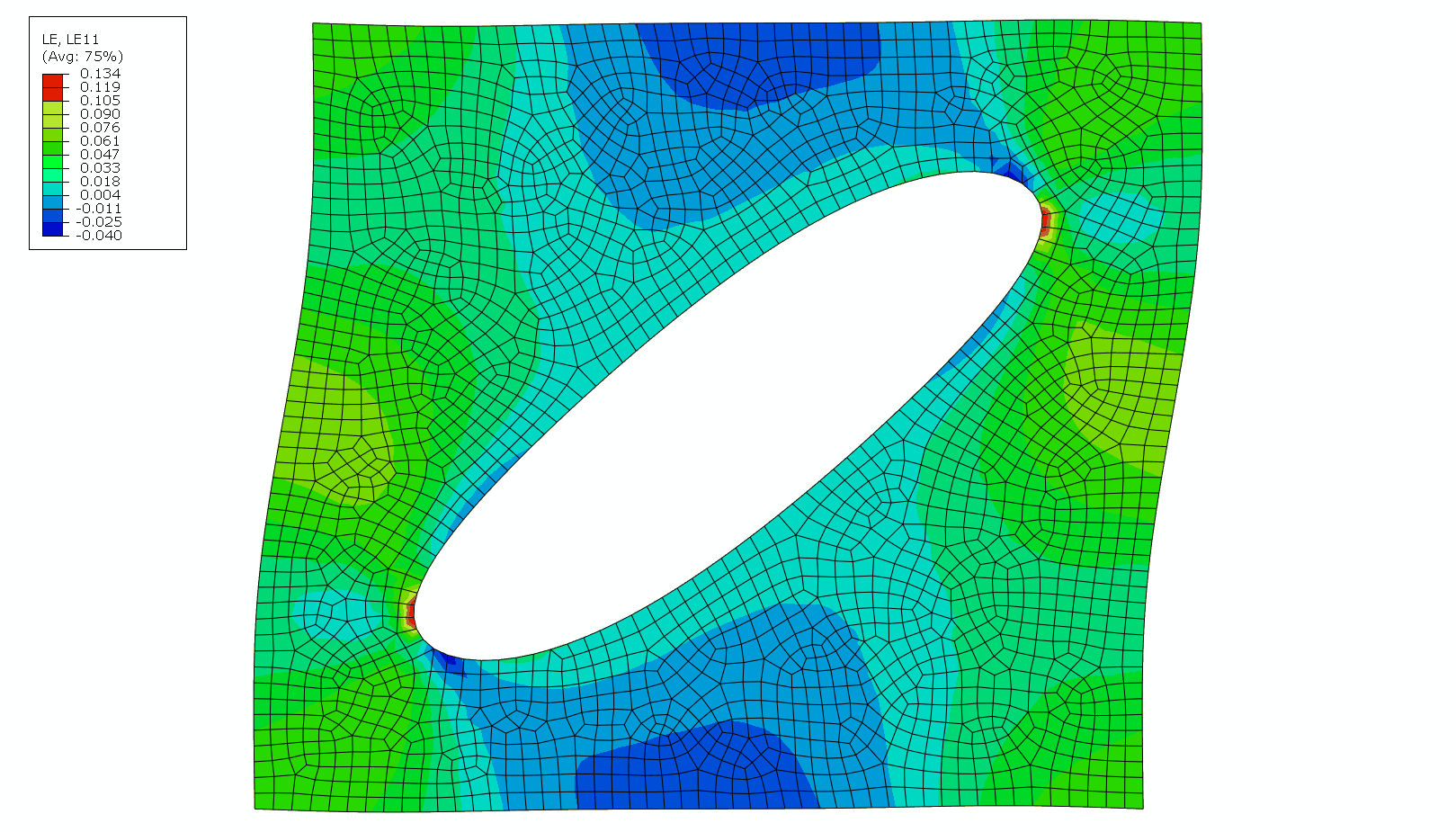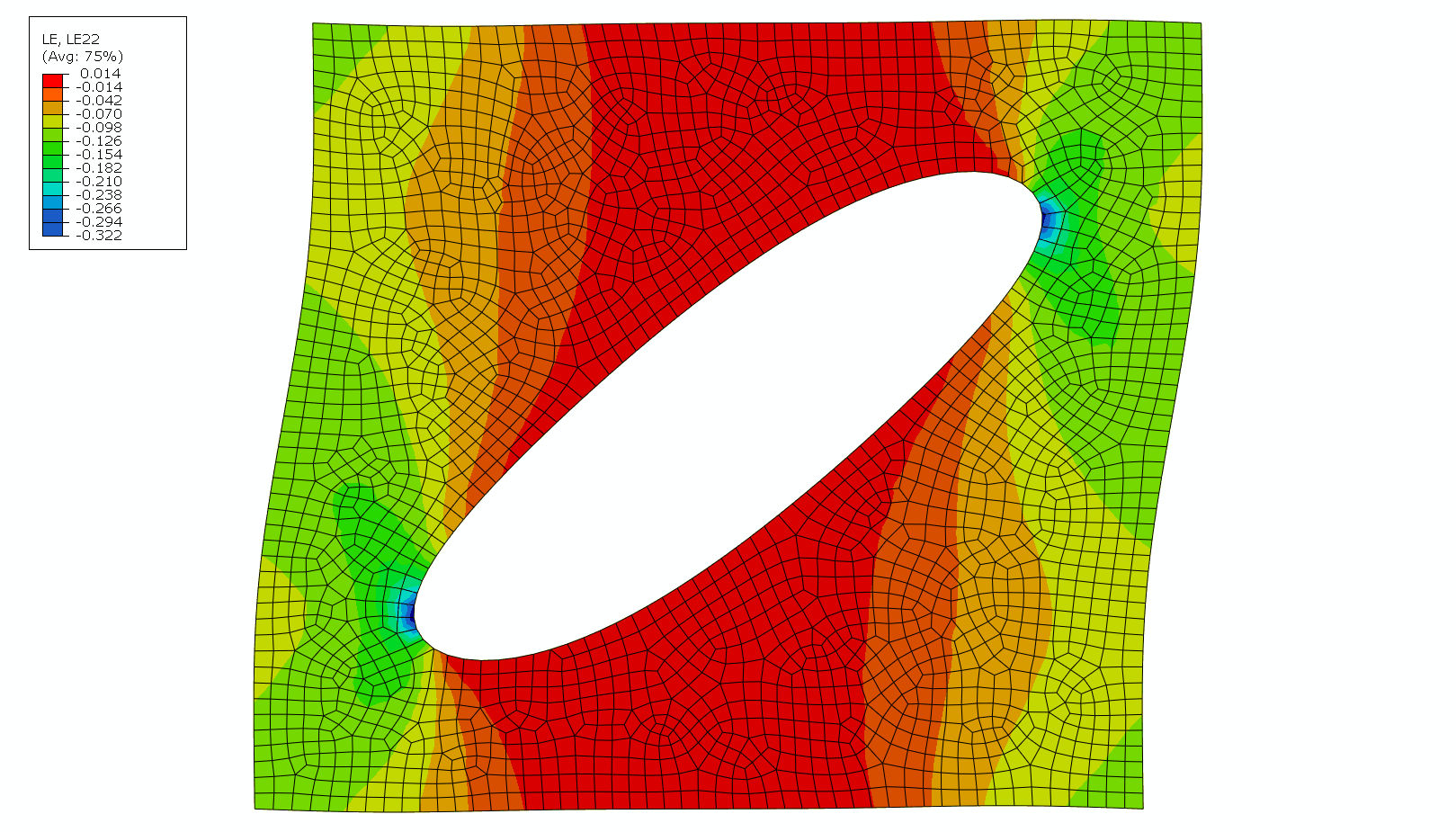周期边界模型的应变